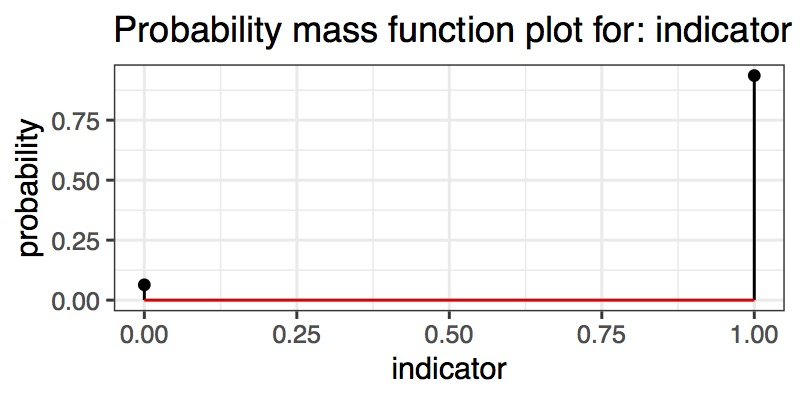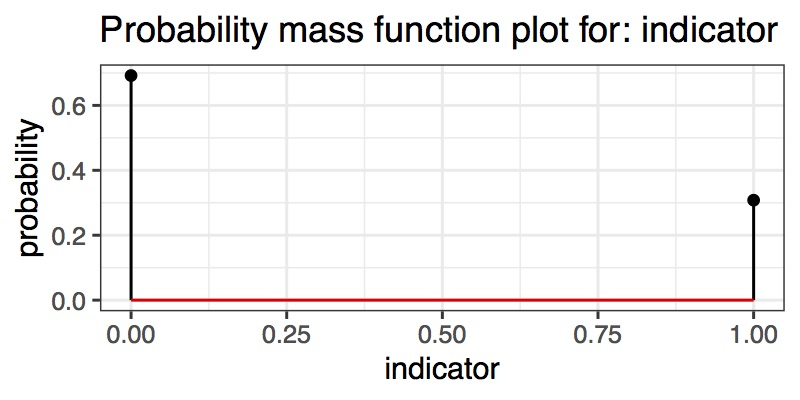# Examples of model selection

• Choice in the likelihood: should we replace the logit transform in the Challenger example by a probit transformation?
• Choice in the prior: in the challenger example, should we replace the normal distribution by a t distribution?
• Variable (covariate) selection: should we use only the temperature covariate, no covariate, or both temperature and humidity, or only humidity, to predict o-ring failure?

# Rabbit holes example

Data

• One patch of land of 1 acre has $$x_1=12$$ rabbit holes.
• A second, disjoint patch of 1 acre has $$x_2=32$$ holes.

Question: do the rabbits prefer one patch over the other?

Models:

• First model: no preference

\begin{align*} \alpha &\sim \text{Exp}(0.1) \\ x_i | \alpha &\sim \text{Poi}(\alpha) \text{ for }i\in \{1,2\} \end{align*}

• Second model: different preferences

\begin{align*} \beta_1 &\sim \text{Exp}(0.1) \\ x_i | \beta_i &\sim \text{Poi}(\beta_i) \text{ for }i\in \{1,2\} \end{align*}

Note: this is a simple example of non-nested models.

# Model selection notation

• $$I$$: an index over a discrete set of models.
• $${\mathscr{Z}}_i$$ for $$i\in I$$: latent space for model $$i$$, i.e. $$z \in {\mathscr{Z}}_i$$ when model $$i$$ is selected.
• $$p_i$$, $$\ell_i$$, $$m_i$$: prior, likelihood, and marginal likelihood densities for model $$i$$, i.e.

$m_i = \int p_i(z) \ell_i(z) {\text{d}}z$

Notation warning: $$m$$ is sometimes called $$Z$$ in the Monte Carlo literature, but here $$Z$$ will be used for the random variable corresponding to the latent variable $$z$$.

Synonyms used for “marginal likelihood”:

• Evidence
• Probability of the data
• Normalization constant

# Computing marginal likehood using PPL

Examples: first two models in Model_selection.html

### Poll: estimate $$m2/m1$$

1. 3.5
2. 0.05
3. 19.3
4. 0.29
5. None of the above

# Key idea for Bayesian model selection

Put a prior $$p$$ on $$I$$, and make the uncertainty over models part of the probabilistic model (let us use uniform priors for today).

The new joint probability density is given by:

$p((i, z), x) = p(i) p_i(z) \ell_i(x | z),$

where $$(i, z)$$ is a member of a new latent space given by:

${\mathscr{Z}}= \bigcup_{i\in I} \left( \{i\} \times {\mathscr{Z}}_i \right),$

Notation: denote the event that model $$i$$ is the model explaining the data by $$M_i$$.

Outcome: Using this construction, model choice can in principle be approached using the same methods as those used in earlier lectures.

### Observations

Graphical modelling: $${\mathscr{Z}}$$ cannot be directly expressed as a non-trivial graphical model (since it is not a product space). How to transform it into a graphical model?

Non-regularity: even with the reductions introduced so far, model selection justifies special attentions because of non-regularities: the likelihood depends in a non-smooth way upon the model indicator variable. Importantly, different models have different dimensionality of the latent space. We will see that MCMC then requires special techniques called trans-dimensional MCMC.

# Bayes factor

Ratio of the marginal likelihood for two models:

$B_{12} = \frac{m_1(x)}{m_2(x)}$

Values of $$B_{12}$$ greater than 1.0 favor model #1 over #2. Values smaller than 1.0 favor #2 over #1. See wikipedia article for some conventions on how to describe the strength of evidence in text: https://en.wikipedia.org/wiki/Bayes_factor.

This is just a reparameterization of the Bayes estimator with an asymmetric 0-1 loss. Note that it is different from a likelihood ratio:

$\frac{\sup_{z_1} \ell_1(x|z_1)}{\sup_{z_2} \ell_2(x|z_2)},$

which is not used within the Bayesian framework.

# Computation of Bayes factor

• In standard Bayesian problems, we need a list of MCMC samples, each of which might be high dimensional.
• Here we only need to compute one scalar for each model, that should be easy… right?
• Surprisingly, this is generally considered harder than getting samples from the posterior distribution.

Many approaches exist, with pros and cons, see for example 19 dubious ways to compute the marginal likelihood of a phylogenetic tree topology

I will outline some key methods:

• Model saturation (today)
• Thermodynamic integration and stepping stone (that’s what you used earlier)
• Sequential Monte Carlo via change of measure (later, if time permits)
• Reversible Jump MCMC (later)

# Model saturation

The idea is to build an augmented model, which can be written as a graphical model, and from which we can still approximate $$m_i(x)$$.

Construction of the auxiliary latent space:

• Instead of defining the global latent space as a union of each model’s latent space, define it as a product space,
• and add to that an indicator $$\mu$$ that selects which model to use to explain the data. The event $$M_1$$ corresponds to $$\mu = 1$$ and $$M_2$$, to $$\mu = 2$$.

This creates the following auxiliary latent space:

${\mathscr{Z}}' = \{1, 2\} \times {\mathscr{Z}}_1 \times {\mathscr{Z}}_2.$

Example: dim($${\mathscr{Z}}_1$$) = 1, dim($${\mathscr{Z}}_2$$) = 2, for the rabbit hole problem

Construction of the auxiliary joint distribution: suppose the current state is $$(\mu, z_1, z_2, x)$$. We need to define an auxiliary joint density $$\tilde p(\mu, z_1, z_2, y)$$.

The idea is that when $$\mu = 1$$, we explain the data $$x$$ using $$z_1$$, and when $$\mu = 2$$, we explain the data $$x$$ using $$z_2$$.

In notation, if $$\mu = 1$$, we set:

$\tilde p(\mu, z_1, z_2, x) = p(\mu) p_1(z_1) p_2(z_2) \ell_1(x | z_1),$

and if $$\mu = 2$$,

$\tilde p(\mu, z_1, z_2, x) = p(\mu) p_1(z_1) p_2(z_2) \ell_2(x | z_2).$

Example: third model in Model_selection.html

Recall:

$m_i = \int p_i(z) \ell_i(z) {\text{d}}z$

1. $$\tilde p(\mu|x) \ge m_{\mu}$$
2. $$\tilde p(\mu|x) \le m_{\mu}$$
3. $$\tilde p(\mu|x) = m_{\mu}$$
4. $$\tilde p(\mu|x) \propto p(\mu) m_{\mu}$$
5. None of the above

Note: in first version of slide, answer 4 was initially written as equal, but answer is proportional, not equal (see next slide)

# Derivation

Let us say $$\mu = 1$$

\begin{align*} \tilde p(\mu|x) &\propto \tilde p(\mu, x) \\ &= \int_{z_1} \int_{z_2} \tilde p(\mu, z_1, z_2, x) \\ &= \int_{z_1} \int_{z_2} p(\mu) p_1(z_1) p_2(z_2) \ell_1(x|z_1) \\ &= p(\mu) \left( \int_{z_1} p_1(z_1) \ell_1(x|z_1) \right) \left( \int_{z_2} p_2(z_2) \right) \\ &= p(\mu) \times m_1 \times 1 \end{align*}

Stepping stone: from stepping stone, we obtained $$\log m_1 \approx -11.94$$ and $$\log m_2 \approx -8.98$$

Model saturation: since $$\mu$$ is Bernoulli, you can find an approximation of $$\tilde p(\mu|x)$$ by looking at the posterior mean of the variable indicator in the third model in Model_selection.html

### Poll: what is the estimate of $$m_2/m_1$$ from model saturation?

1. 0.95
2. 0.05
3. 19
4. 1/19
5. None of the above

# Implicit penalty for model complexity

Consider now the final exercise in Model_selection.html.

### Poll: what model is selected if the observations are $$(12, 13)$$?

1. Model 1 (simpler one)
2. Model 2 (more complicated one)

# Implicit penalty for model complexity

• Old plot for $$(12, 32)$$• New plot for $$(12, 13)$$# Model saturation, pros and cons

Pro: can use graphical-model based MCMC methods that do not have marginal likelihood computation methods.

Cons:

• Limited to finite collections of models, $$|I| < \infty$$.
• Computational cost does not scale well in $$|I|$$ (but tricks can relax this, consider for example spike-and-slab example, where an exponential family of models can be efficiently represented by “sharing” latent variables across models)

Chapter 7 in Model Choice, except for section 7.3.

# Optional exercise

• Apply the material we have seen so far, in particular, PPLs, hierarchical models, and model selection, to estimate the vaccine efficacy of the following 3 trials:
trials,arms,groupSizes,numbersOfCases
AZ-Oxford (combined),vaccinated,5807,30
AZ-Oxford (combined),control,5829,101
Pfizer-BioNTech,vaccinated,18198,8
Pfizer-BioNTech,control,18325,162
Moderna-NIH,vaccinated,14134,11
Moderna-NIH,control,14073,185
• Hints:
• use a model of the form ~ Binomial(groupSize, incidence * (1.0 - efficacy)) where efficacy is 0.0 for control, and random for each vaccine
• use the following template below, so that data is imported automatically when using the command line arguments --model.data data/vaccines/data.csv
model Vaccines {

param GlobalDataSource data

param Plate<String> trials
param Plate<String> arms
param Plated<IntVar> groupSizes
random Plated<IntVar> numbersOfCases

random Plated<RealVar> efficacies
random Plated<RealVar> incidences

random RealVar a ?: latentReal, b ?: latentReal, c ?: latentReal, d ?: latentReal

laws {

// fill this

}
}